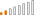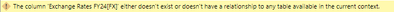cancel
Showing results for
Did you mean:

Fabric is Generally Available. Browse Fabric Presentations. Work towards your Fabric certification with the Cloud Skills Challenge.Helper II

## SUMX difficulty: Does not perform as expected in the total

I've been having difficulty using SUMX in a particular situation and I've reduced the complexity of the model almost to nothing and I still can't get the expected result.

I have a table of fixed exchange rates consolidating to a single currency, and a list of transactions with their currency recorded.  You can see they have a different column name for the two, so the use of columns in a visual later is unambiguous:I want to calculate the consolidated value:

``````ExpensesToCommonCurrency =
SUMX(VALUES('Exchange Rates FY24'[Local Currency]),
DIVIDE(SUM(Expenses[transactionamount]) , SELECTEDVALUE('Exchange Rates FY24'[FX]), BLANK())
)``````

which works with 'Exchange Rates FY24' context, but not in aggregate (showing rows with no data):I have tried changing SELECTEDVALUE to MAX or MIN but in each case it is completely losing the 'Exchange Rates FY24' context from the SUMX and just calculating the max or min across the entire XR table.

Edit:  If I switch to SUMX('Exchange Rates FY24', [Expression]) then I get double the expected value for EUR and CHF, despite the fact that the FX column has no direct relationship with the Expenses table at all.  And the aggregate is still blank.

What am I overlooking?

Greg

1 ACCEPTED SOLUTIONHelper II

Found the solution.  In the aggregate, the SUMX context is not passed to sum(transactionamount) unless it is wrapped in a "CALCULATE".  I don't know what the context is if you don't wrap it, but this works:

``````ExpensesToEUR =
SUMX(VALUES('Exchange Rates FY24'[FX]),
DIVIDE(CALCULATE(SUM(Expenses[transactionamount])) , 'Exchange Rates FY24'[FX])
)``````

Side note, I was struggling with a similar problem where table A filters table B and C, and I need to calculate something using B with a lookup of a value in C.  SELECTEDVALUE on Table C was not working within a SUMX.  Wrapping it in a CALCULATE is the likely answer.

3 REPLIES 3Helper II

Found the solution.  In the aggregate, the SUMX context is not passed to sum(transactionamount) unless it is wrapped in a "CALCULATE".  I don't know what the context is if you don't wrap it, but this works:

``````ExpensesToEUR =
SUMX(VALUES('Exchange Rates FY24'[FX]),
DIVIDE(CALCULATE(SUM(Expenses[transactionamount])) , 'Exchange Rates FY24'[FX])
)``````

Side note, I was struggling with a similar problem where table A filters table B and C, and I need to calculate something using B with a lookup of a value in C.  SELECTEDVALUE on Table C was not working within a SUMX.  Wrapping it in a CALCULATE is the likely answer.Continued Contributor

Hi Greg,

I would just divide the sum of Expenses[transactionamount] by the exchange rates, and the measure amount below is already in converted into EUR amount.I don't think you need sumx to achieve what you need to do.Helper II

This gives me an errorbut I found the solutionAnnouncements#### Power BI Monthly Update - November 2023

Check out the November 2023 Power BI update to learn about new features.#### Fabric Community News unified experience

Read the latest Fabric Community announcements, including updates on Power BI, Synapse, Data Factory and Data Activator.#### The largest Power BI and Fabric virtual conference

130+ sessions, 130+ speakers, Product managers, MVPs, and experts. All about Power BI and Fabric. Attend online or watch the recordings.Top Solution Authors
Top Kudoed Authors
Users online (1,472)